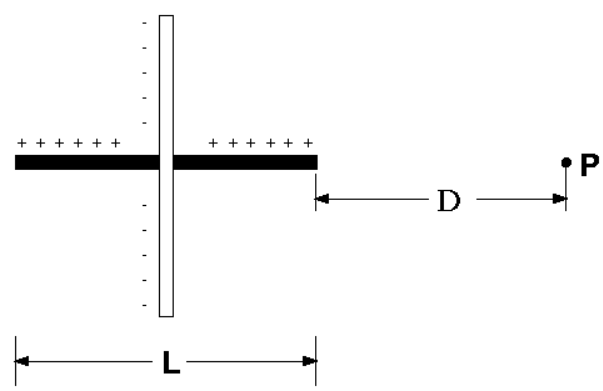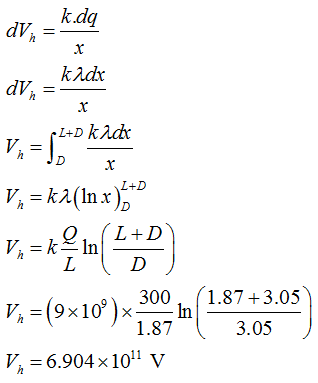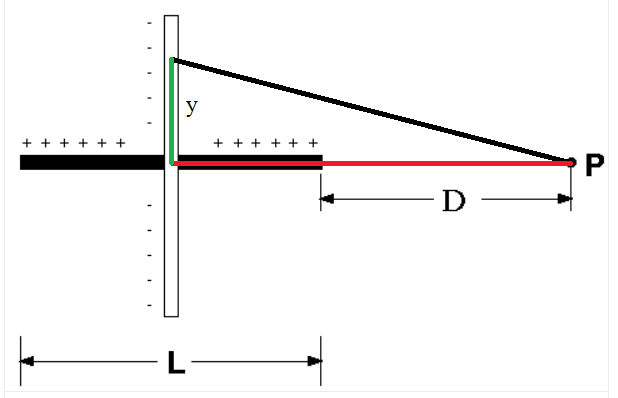Question
1 views

Two rods of equal length L = 1.87 m form a symmetric cross. The horizontal rod has a charge of Q = 300.0 C, and the vertical rod a charge of -Q = -300.0 C. The charges on both rods are distributed uniformly along their length. Calculate the potential at the point P at a distance D = 3.05 m from one end of the horizontal rod.help_outlineImage Transcriptionclose+ + + + + + + + + + + + -D P. fullscreen
check_circle

star
star
star
star
star
1 Rating
Step 1

Given:

the length of rod (L) = 1.87 m

charge on horizontal rod (Q1 ) = 300 C

charge on vertical rod (Q2 ) = -300 C

distance between horizontal plate and point P (D) = 3.05 m

Step 2

charge density of rod = Q/L

potential at point P due to horizontal rod is,Step 3

distance between any point on rod from point P is hypotenus,...

### Want to see the full answer?

See Solution

#### Want to see this answer and more?

Solutions are written by subject experts who are available 24/7. Questions are typically answered within 1 hour.*

See Solution
*Response times may vary by subject and question.
Tagged in

### Physics